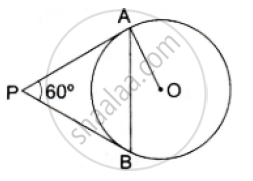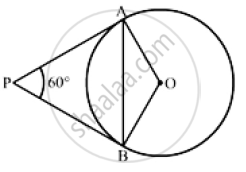Share
Notifications

View all notifications
Books Shortlist
Your shortlist is empty

# In the Given Figure, Pa and Pb Are Two Tangents to the Circle with Centre O. If ∠Apb = 60° Then Find the Measure of ∠Oab. - CBSE Class 10 - Mathematics

Login
Create free account

Forgot password?
ConceptCircles Examples and Solutions

#### Question

In the given figure, PA and PB are two tangents to the circle with centre O. If ∠APB = 60°  then find the measure of ∠OAB.#### Solution

Construction: Join OBWe know that the radius and tangent are perpendicular at their point of contact

∵ ∠OBP = ∠OAP = 90°
Now, In quadrilateral AOBP
∠AOB + ∠OBP + ∠APB +∠OAP = 360°        [Angle sum property of a quadrilateral]
⇒ ∠AOB +90° + 60° + 90° = 360°
⇒ 240° + ∠AOB = 360°
⇒ ∠ AOB = 120°
Now, In isosceles triangle AOB
∠AOB  + ∠OAB + ∠OBA = 180°                [Angle sum property of a triangle]
⇒ 120° +  2 ∠OAB =180°               [∵ ∠OAB = ∠OBA]
⇒  ∠OAB = 30°

Is there an error in this question or solution?

#### Video TutorialsVIEW ALL 

Solution In the Given Figure, Pa and Pb Are Two Tangents to the Circle with Centre O. If ∠Apb = 60° Then Find the Measure of ∠Oab. Concept: Circles Examples and Solutions.
S